Summary of Le Chatelier's Principle

Note Le Chatelier's principle- If a change is imposed on a chemical system at equilibrium, the system will react in a way so as to re-establish equilibrium in such a way as to partially undo the change.

We will summarise Le Chatelier's Principle using the reaction below

N2(g) + 3H2(g)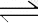2NH3(g) ΔH = -92 kJ/mol

Changes to the equilibrium can occur in the following ways.

### Increasing the mass of a species

Hydrogen gas is added to it.

• To oppose the change, some of the added hydrogen must be removed.
• The equilibrium position will shift to the right to absorb some of the hydrogen.
• The rate of the forward reaction is now greater than the rate of the reverse reaction. This continues until equilibrium is achieved, where at this point, both the forward and backward rates of reaction are equal.
• Notice how in the new equilibrium the rates of the backward and forward reactions, although equal, are higher than during the previous equilibrium.When equilibrium is reached once more notice how :

• The concentration of hydrogen gas is greater than before as the change is only partially undone. To oppose the change, some of the added hydrogen must be removed.
• The value of K is constant
• The changes in concentration are equal to the molar ratios given in the balanced equation. For example, [N2] decreases by a third that of the decrease in [H2] . The increase in [NH3] is 2/3 that of the decrease in [H2]### Decreasing concentration

Using the same equilibrium system, as above, but in this case removing H2 , lowers the concentration of hydrogen gas..

• Since there is a decrease in [H2], the system responds by producing H2 molecules.
• The equilibrium system shifts to the left creating more H2.
• The rate of the reverse reaction now increases more than the forward reaction, since the concentration of reactants has decreased.
• The rates of the forward and backward reactions, although equal, are lesser than before as the concentrations of H2 and NH3 decrease overall.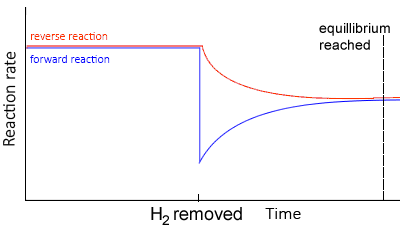At equilibrium,

• Since the change is only partially opposed the concentration of H2 would be less than at the first equilibrium position of the system.
• The value of Kc remains the same, in other words it is constant (Kc)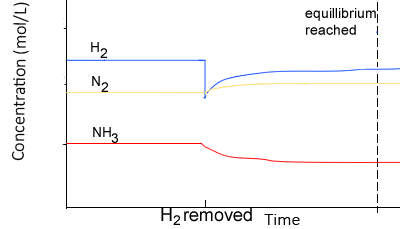### Change in volume

Decreasing the volume of a gaseous system will increase the pressure. Increasing the pressure, according to Le Châtelier’s principle, will promote a shift to the right to reduce the number of particles. and hence lower the pressure.

• The system moves so as to reduce the total pressure.
• This is done by reducing the total amount of particles in the system, thus shifting the equilibrium position towards the side with the least particles. The side with more particles will experience a greater increase in concentration , due to a volume decrease, hence more of those particles would react.
• The rates of the backward and forward reactions are equal, but greater than before.At equilibrium,

• At the new equilibrium the concentrations of all particles will increase overall as the change is partially opposed.
• Value of K remains the same, in other words it is constant (Kc)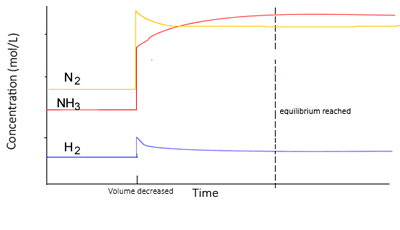Increase in volume

If the volume of the reaction vessel is increased, pressure decreases, and the system shifts in the direction of most particles thus increasing the total pressure

At equilibrium, the concentration of all particles will be lower than before the change as change is partially opposed.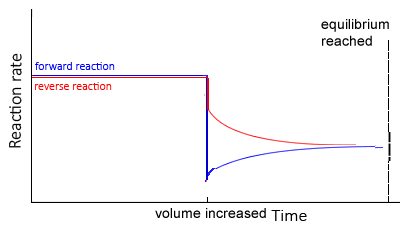Note that the equilibrium position will not change if:
- a pressure change is due to the addition of an inert gas. It will only change if the pressure change is due to a change in volume .

or

- the reaction has equal particles on both sides.
For example
The gaseous equilibrium H2(g) + Cl2(g)2HCl (g) has two particle on both the reactants and products side. It cannot respond to a change in pressure due to volume change. The reaction, 3H2(g) + N2(g)2NH3 (g), however, can respond as there are four particles on the reactants side and two particle on the products side.

### Change in temperature

The heat of the reaction must be known so that we can predict the response of the system to temperature change.

In the reaction below, the forward reaction is exothermic while the reverse reaction is endothermic.

N2(g) + 3H2(g)2NH3(g) ΔH = -92 kJ/mol

Since temperature has changed, so does the equilibrium constant.

 If the forward reaction is exothermic, increasing temperature decreases Kc, because it drives the reaction in the backward direction in order to absorb the heat energy. Decreasing temperature, however, increases Kc as the system shifts to the right..
 If the forward reaction is endothermic, increasing temperature increases the value of Kc and decreasing temperature lowers the value of Kc.According to Le Chatelier’s principle

• If an equilibrium system is cooled, the system will adjust by creating heat by favouring the exothermic reaction
• Thus, the concentration of the products of the exothermic reaction would increase, while the concentration of the reactants would decrease
• If an equilibrium system is heated the system will adjust by using heat and favouring the endothermic reaction
• Thus, the concentration of the products of the endothermic reaction would increase, while the concentration of the products would decrease### Presence of a catalyst

• When a catalyst is added, it lowers the activation energy and increases the rate of reaction by providing an alternate reaction pathway.
• When a catalyst is added to a system not in equilibrium, equilibrium is reached faster while the equilibrium constant(K) and the amount of product formed remain unchanged..
• If a catalyst is added to a system in equilibrium, the system remains in equilibrium as the catalyst speeds up the forward and reverse reactions equally.

1)    Consider the following system
2 NO2 (g)N2O4 (g) ΔH = -92 kJ/mol

NO2 gas is placed in a sealed vessel and reacts according to the equation above. The system is allowed to reach equilibrium.
a) What is the equilibrium constant at t1? Solution

b) At t2 the temperature of the reaction vessel is increased and allowed to reach equilibrium once again. Draw what the graph looks like after t2. Solution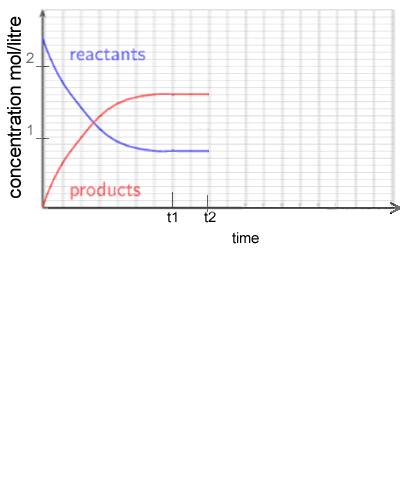c) Complete the graph on the right as temperature is increased at t2.

Solution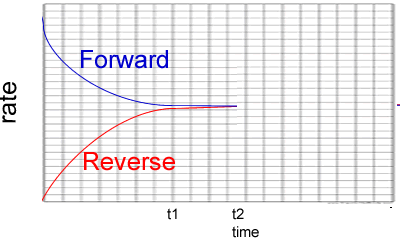2) Consider the graph on the right. It represents the reactions below.
A(g) + B(g)AB(g) ΔH = negative kJ/mol
a) What can you say about the system between t1 and t2?

Solution
b) Which of the following is a plausile change to the system at t2? Explain why you selected or did not select each option.

• An increase in concentration of the product. Solution

• An in increase in temperature Solution

• A change in pressure due to a volume decrease. Solution

• The addition of a catalyst . Solution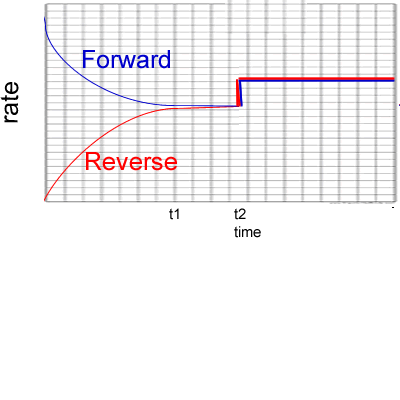3) Reactants A and B are placed in a 2.00 L sealed reaction vessel and allowed to reach equilibrium. The reaction is given below.
A2(g) + 2B2(g)2AB2 (g) ΔH = positive kJ/mol

a) Calculate the equilibrium constant at the :

- 4 minute mark
Solution

- 10 minute mark
Solution

b) What happened at the 6 minute mark? Solution

c) At the 11 minute mark substance X is added to the system. Substance X reacts with AB2 to form a solid compound. Draw, on the graph on the right, how the system will react. Solution

4) What are the advantages and disadvantages of increasing the temperature of an equilibrium system involving an exothermic reaction? Solution

5) How can the disadvantages be overcome in question 4) above? Solution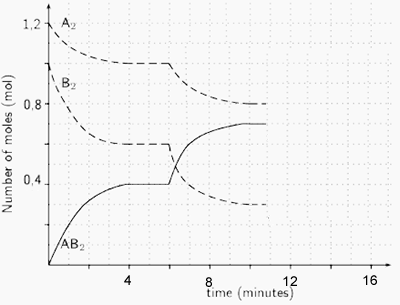Continue with an exercise of relating rates and concentration changes as a system's response to stress.